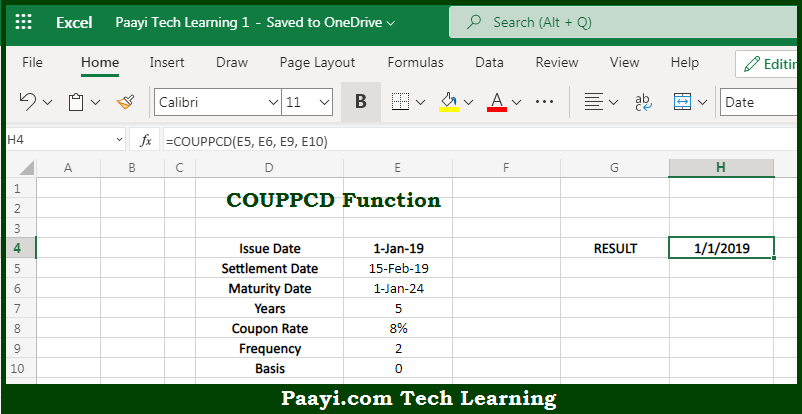# Learn How to Use Microsoft Excel COUPPCD Function

Written by | 0 Comments | 577 Views

In this article, you will learn how to use the Microsoft Excel COUPPCD function and its prime function in Microsoft Excel. You will also get to know the Microsoft Excel COUPPCD function return value and syntax with the help of some examples.

Microsoft Excel COUPPCD Function

The main purpose of the Microsoft Excel COUPPCD function is to get the previous coupon date before the settlement date. That implies, with the help of the COUPPCD function you can able to return the previous coupon date before the settlement date for a coupon bond. So, with the help of the COUPPCD function, you can able to get the previous coupon date before the settlement date.

Return Value of COUPPCD Function

The return value will be the previous coupon date.

Syntax of COUPPCD Function

=COUPPCD(settlement, maturity, frequency [basis])

Where the arguments:

• settlement: This is the settlement date of the security.
• maturity: This is the maturity date of the security.
• frequency: This is the number of coupon payments per year, where annual = 1, semi-annual = 2, and quarterly = 4.
• basis: This is the day count basis, default is 0 (optional).

How to Use Microsoft Excel COUPPCD Function?So we know that Microsoft Excel COUPPCD function you can able to get the previous coupon date before the settlement date. That implies, with the help of the COUPPCD function you can able to return the previous coupon date before the settlement date for a coupon bond. So, with the help of the COUPPCD function, you can able to get the previous coupon date before the settlement date.